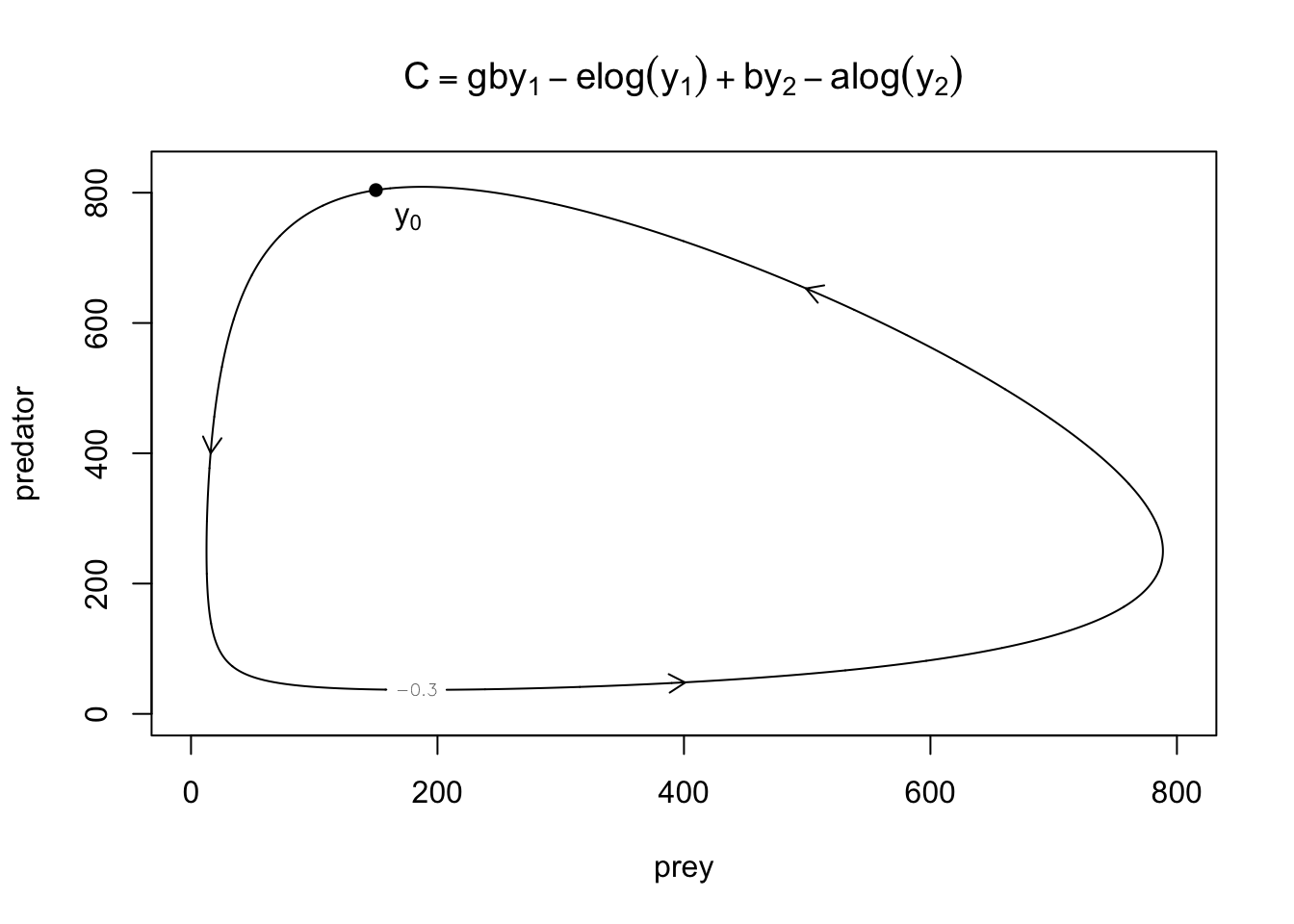These notes are based on Chapter 13 of Jones, O., Maillardet, R., & Robinson, A. (2014). Introduction to scientific programming and simulation using R (2nd ed.). CRC Press.

# Introduction

Recall, from your calculus class, that solving a system of ordinary differential equations (ODEs) $\frac{d}{dt}\mathbf{y}(t) = \mathbf{f}(\mathbf{y}(t))$ means finding a (vector-valued) function $$\mathbf{y}(t)$$ that satisfies the system of equations. The following is a famous model based on the Lotka-Volterra equations.

In differential equations, notations can easily get confusing. In these notes, I explicitly use boldface to denote vectors and vector-valued functions (e.g., $$\mathbf{y}$$ and $$\mathbf{f}$$).

In these notes, we consider only autonomous systems, that is, the right-hand side depends on $$t$$ only through $$\mathbf{y}(t)$$.

## Predator–prey model

The predator-prey model is used to describe the population dynamics of two species, one as a predator and the other as a prey. The model consists of a pair of ODEs: \begin{align} \frac{dy_1}{dt} &= ay_1 - by_1y_2\\ \frac{dy_2}{dt} &= gby_1y_2 - ey_2 \end{align} where

• $$y_1$$ : prey population
• $$y_2$$ : predator population
• $$a$$ : natural growth rate of prey in the absence of predation
• $$b$$ : death rate due to the predation
• $$g$$ : birth rate of predator due to the captured prey
• $$e$$ : natural death rate of predator.

The system is known to have periodic solutions, which do not allow simple expressions. Fortunately, it is still easy to obtain an implicit equation of $$y_1$$ and $$y_2$$.

Eliminating $$dt$$ from the above system of ODEs, we have: \begin{alignat}{2} &&(ay_1-by_1y_2)dy_2 &= (gby_1y_2-ey_2)dy_1\\ &\Leftrightarrow\quad &\int \frac{a-by_2}{y_2}dy_2 &= \int \frac{gby_1-e}{y_1}dy_1\\ &\Leftrightarrow\quad & C &= gby_1-e\log(y_1)+by_2-a\log(y_2) \end{alignat} where $$C$$ is a constant.

With $$a=0.05$$, $$b=0.0002$$, $$g=0.8$$, $$e=0.03$$, and $$C=-0.3$$, we have the following orbit in a phase space.

a <- 0.05
b <- 0.0002
g <- 0.8
e <- 0.03
C <- -0.3
y1 <- seq(0, 800, length=1000)
y2 <- seq(0, 830, length=1000)
out <- outer(y1, y2, function(y1,y2) g*b*y1 - e*log(y1) + b*y2 - a*log(y2) )
contour(y1, y2, out, levels=C, xlab="prey", ylab="predator")
from_x <- c(16.05, 400, 500)
from_y <- c(401, 48, 652.3)
to_x <- c(16, 401, 499)
to_y <- c(400, 48.1, 653)
y0 <- c(150, 804)
points(y0, y0,pch=16)
text(y0, y0-50, expression(y), pos=4)
arrows(from_x, from_y, to_x, to_y, length=.1)
title(expression(C == g*b*y - e*log(y) + b*y - a*log(y)))Just as we can find closed-form antiderivatives only in special integration problems, we can find analytical solutions only for some types of ODEs. Again, numerical methods allow us to (approximately) solve a wider class of problems.

## Numerical solutions

As nonsensical as it sounds, if we knew $$\mathbf{y}(t)$$ on the right-hand side of the above ODEs, a solution would be a usual integral with respect to $$t$$: $\mathbf{y}(t) = \int \mathbf{f}(\mathbf{y}(t))dt .$ But, of course, we do not know $$\mathbf{y}(t)$$, which is the point of this exercise.

Nevertheless, when we work on an initial value problem: $\mathbf{y}(h) = \mathbf{y}(0) + \int_0^h \mathbf{f}(\mathbf{y}(t))dt ,$ such a “nonsensical” integral gives us a reminder that solving a differential equation is doing integration.

Almost always, whether it is analytical or numerical integration, when it is hard, we approximate the integrand by a simple function.

In analytical integration, an integral is defined as a limit. So, it does not matter whether we approximate the integrand by a function or constant values. In numerical integration, on the other hand, the choice of simple functions affects the performance of approximation because we always have to settle for finite granularity depending on our computational capacity.

Recall the technique used in Numerical Integration, where we approximate a complex function over each subinterval by simple functions so that we can carry out an integral with ease. Specifically, linear functions in the trapezoidal rule and quadratic functions in Simpson’s rule. Why not applying the same idea here — approximating an unknown function $$\mathbf{y}(t)$$?

Besides the similarity, also note the difference. In the standard numerical integration, we can evaluate the function wherever we like. In contrast, here, we can evaluate only the derivative $$\frac{d\mathbf{y}}{dt} = \mathbf{f}$$ and evaluation points $$\{\mathbf{y}(t)\}$$ themselves depend on our repeated approximation.

# Euler’s method

Let’s try linear approximation. Let $$\hat{\mathbf{y}}(t)$$ be approximation to $$\mathbf{y}(t)$$ for $$t>0$$, and $$\hat{\mathbf{y}}(0) = \mathbf{y}(0)$$. If $$\mathbf{y}(t)$$ is linear, $$\mathbf{f}(\mathbf{y}(t)) = \frac{d}{dt}\mathbf{y}(t)$$ is constant. A natural choice is $$\mathbf{f}(\mathbf{y}(t)) = \mathbf{f}(\mathbf{y}(0))$$ for $$t\in[0,h]$$, and we can easily compute $$\mathbf{f}(\mathbf{y}(0))$$ using the knowledge of $$\mathbf{y}(0)$$ and $$\mathbf{f}$$. Thus, \begin{align} \hat{\mathbf{y}}(h) &= \hat{\mathbf{y}}(0) + \int_0^h \mathbf{f}(\hat{\mathbf{y}}(0))dt\\ &= \hat{\mathbf{y}}(0) + h\mathbf{f}(\hat{\mathbf{y}}(0)) \end{align}

If we are happy to make repeated approximation, then we have Euler’s method for solving ODEs: \begin{align} \hat{\mathbf{y}}(nh+h) &= \hat{\mathbf{y}}(nh) + \int_{nh}^{nh+h} \mathbf{f}(\hat{\mathbf{y}}(nh))dt\\ &= \hat{\mathbf{y}}(nh) + h\mathbf{f}(\hat{\mathbf{y}}(nh)) \end{align} for $$n\in\{0,1,\dots\}$$.

Approximation errors $$\|\hat{\mathbf{y}}(nh) - \mathbf{y}(nh)\|$$ are accumulated over time.

Let’s illustrate it using the predator–prey model. Each process starts at $$\mathbf{y}_0=(150, 804)$$, which is a point on the orbit.

LV <- function(y) c(a*y - b*y*y, g*b*y*y - e*y)
euler <- function(f, y0, h, N) {
Y <- matrix(nrow=N+1, ncol=length(y0)) # empty matrix
Y[1,] <- y0 # R has 1-base indexing. Y[1,] for y0.
for (n in 1:N) {
Y[n+1,] <- Y[n,] + h*f(Y[n,])
}
return(Y) # returned value is a matrix
}
for (h in c(1, 0.1)) {
N <- 880/h
Y <- euler(LV, y0, h, N)
plot(Y[,1], Y[,2], type='l', xlab="prey", ylab="predator")
points(Y[1,1], Y[1,2])
text(Y[1,1]+10, Y[1,2], expression(y), pos=1)
arrows(Y[N+1,1], Y[N+1,2],
Y[N+1,1]+LV(Y[N+1,]), Y[N+1,2]+LV(Y[N+1,]),
length=.1, lwd=2)
title(paste("Euler's method (h = ", h, ")", sep=""))
}